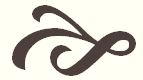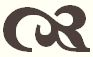#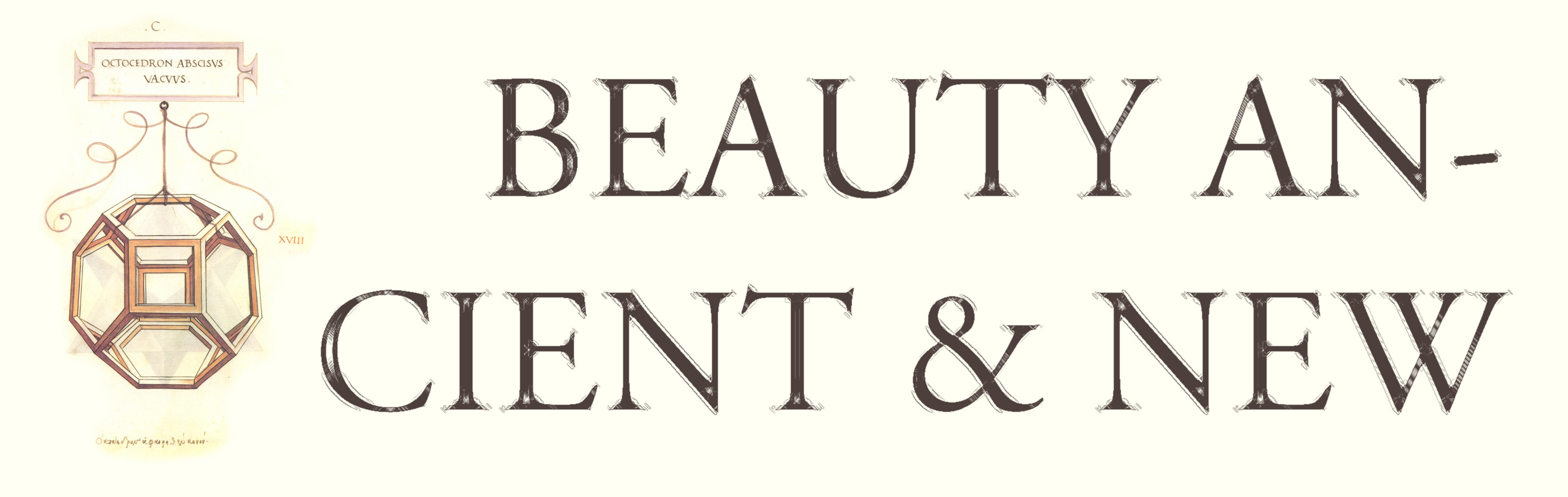Fractal Art

At one time I was an art student; however, they showed us a film on fractal geometry in a design class, and I went out forthwith and changed my major to mathematics. (Actually it wasn't quite forthwith but these are matters of the heart. I still continue to paint; you can view some of my recent paintings and drawings here.) Eventually I went into a completely different field of math, but fractal geometry still captivates my imagination.

Most of the fractals below are described in Benoit Mandelbrot's seminal work, The Fractal Geometry of Nature. I created the underlying figures using GeoGebra (free dynamic geometry software), writing a recursive code for each. I then imported the layers into Photoshop one at a time and used them as stencils, blending them with the beautiful rights-free textures available at Lost & Taken.

Additional information on fractals and creating fractal art is forthcoming. For the time being, you can view my interactive explorations via my profile page at GeoGebraTube, and my GeoGebra images on my blog. Here, rather than explaining what a fractal is, I shall content myself with the following quatrain from Alexander Swift, and then proceed to the pictures:

So, Nat'ralists observe, a Flea,
Hath smaller Fleas that on him prey,
And these have smaller Fleas to bit 'em,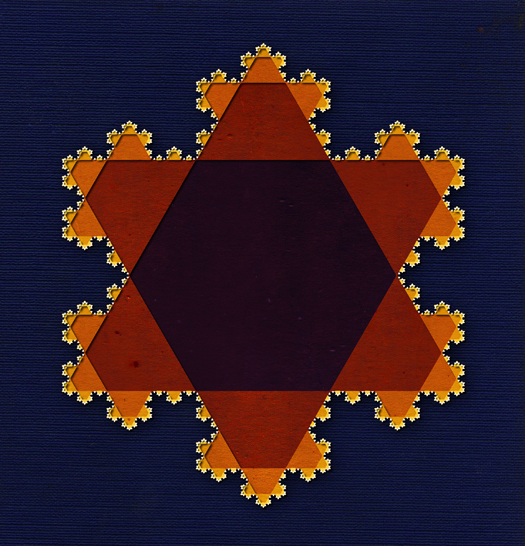The Koch snowflake, named for the Swiss mathematician Helge von Koch (1870 – 1924) who first described it, is the quintessential fractal. Starting with an equilateral triangle, trisect each side and erect a smaller equilateral triangle upon the middle segment. Do the same for each of the twelve segments that result, and continue in this way, ad infinitum. The snowflake that results is a figure with a finite area and an infinite perimeter. Mandelbrot calls it a Koch island, with the idea that this regular figure, infinite in its devious turnings and displaying the same degree of complexity at all scales, is a model for irregular coastlines.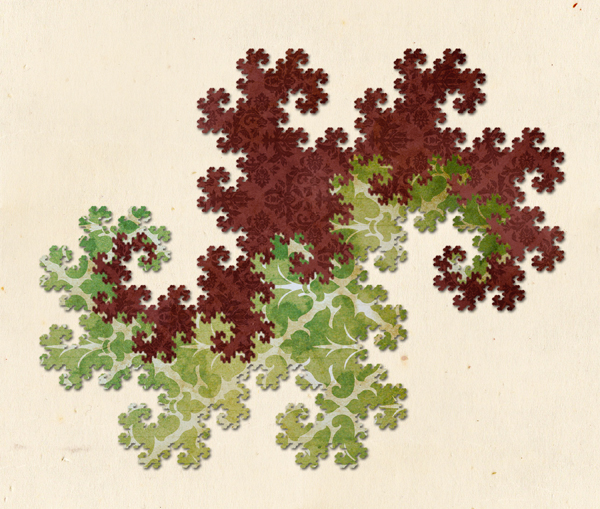Each iteration in the recursive construction of the Harter-Heighway dragon sweep (named for the NASA scientists who first investigated it) can, in principle, be formed by repeatedly folding a long strip of paper in half. The sweep an example of a space-filling curve (see below). Its boundary, known as a dragon curve, is a fractal; it is composed of an infinite sequence of similar shapes joined by wasp-waists. Dragon sweeps can be used to tile the plane, and the pair of juxtaposed sweeps shown above is called a twindragon. The dragon sweep was made famous by being depicted on the title pages of Jurassic Park.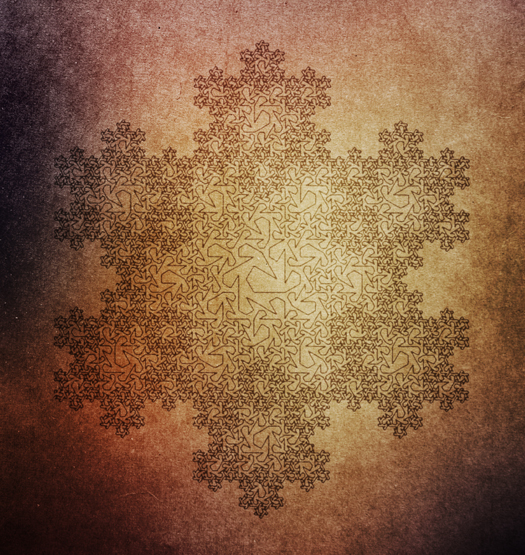Plane-filling curves like the dragon sweep were discovered in the late nineteenth century and caused great consternation in the mathematical community, showing as they did that a planar or solid region can be "filled in" by an infinitely thin, winding curve. The first continuous curve was described by Guiseppe Peano (1858 – 1932), who'd been inspired to look for one by Georg Cantor's findings in set theory. A better known example was discovered by David Hilbert (1862 – 1943). These curves fill a square; the one shown above fills a Koch snowflake. The first iteration consists of a seven-segment polygonal curve connecting points on the trisected sides of an equilateral triangle. Each segment is then replaced by a copy of the original figure, and so on, ad infinitum. This construction was designed by Mandelbrot and described in his Fractal Geometry of Nature.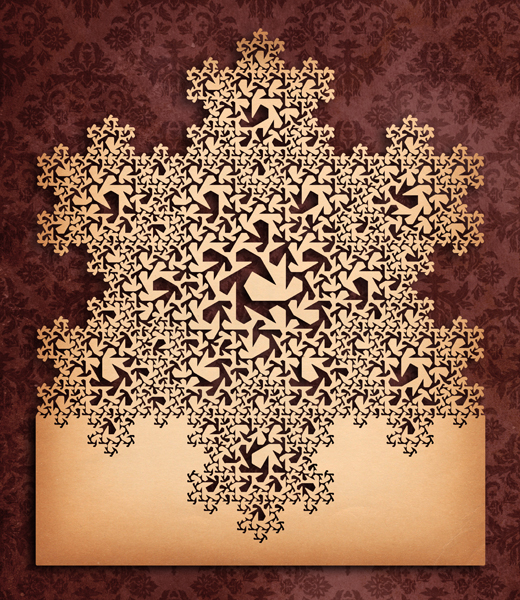Filling in the plane on one side of the snowflake-filling Peano curve above forms what Mandelbrot calls an advanced teragon (from tera-, or "monster," and –gon, or "side"). As he puts it: "This advanced teragon, shown as boundary between two fantastically intertwined domains serves better than any number of words to explain what plane-filling means."Though considered by some to be a fractal, the Pythagoras tree doesn't meet the usual criteria. It is self-similar like many fractals, however. It's constructed by erecting squares on the three sides of a right triangle (as in Euclid's proof of the Pythagorean Theorem). Similar triangles are erected on the opposite sides of the smaller squares, and the process is repeated, ad infinitum. The Pythagorean Theorem implies that the sum of the squares at each level (all squares of a single color) has the same area as the base square.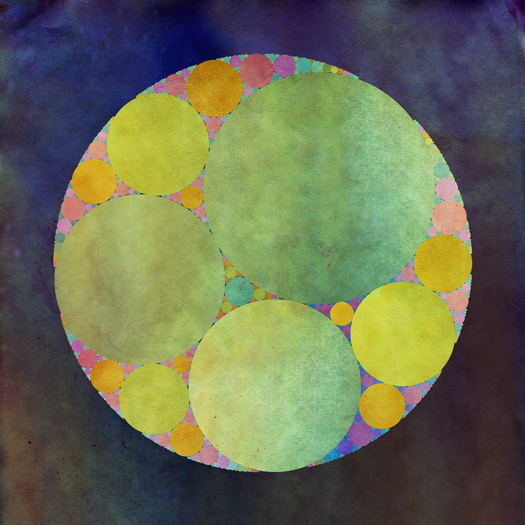The great Greek geometer Apollonius of Perga developed an algorithm which, given three mutually tangent circles (the large green circles), constructs two other circles tangent to the three (the blue-green circle at the center, and the large circle bounding the other four). The construction of the "inner" circle can then be repeated for each of the six circular "triangles" that result, and so on, ad infinitum. This process produces an Apollonian net; the figure contained by each "triangle" is called an Apollonian gasket.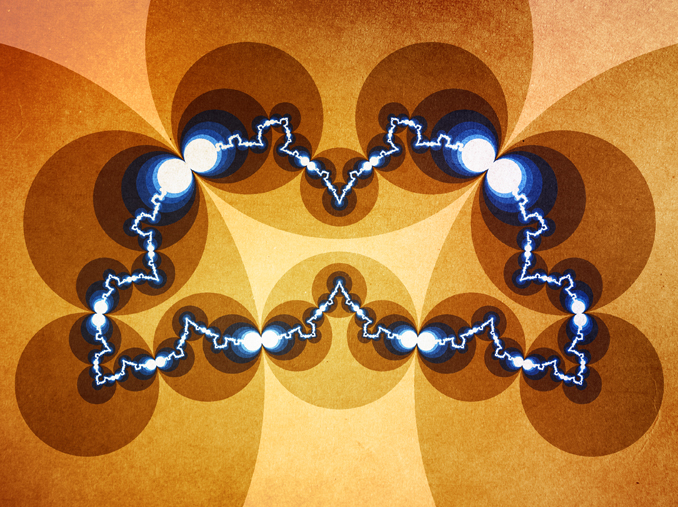Beginning with a Poincaré chain of four circles (a ring of four circles, each of which is tangent to its neighbors), reflect each triple across the remaining circle. (This "reflection" is what is usually called inversion; it is a way of turning the plane inside-out across the circle, with the center going to infinity and infinity going to the center.) This produces a Poincaré chain of twelve circles. Repeat the process, reflecting the chain across each of the original circles, resulting in thirty-six circles, and so on, ad infinitum. The fractal curve that results is a closed Jordan curve, separating the plane into two disjoint sets, and is infinitely jagged, with tangent lines at no points. I call it a Poincaré necklace.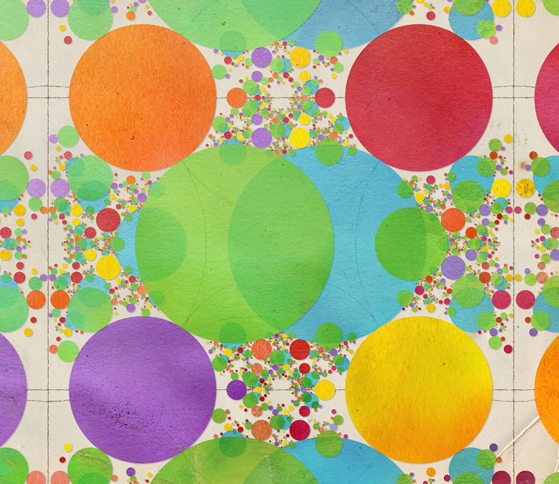Begin with a Poincaré chain of four circles with centers at the vertices of a rhombus, and a rectangle of lines, each of which passes through the center of a circle; these are shown faintly in the background. Construct the six colored circles so that each is orthogonal to a triple of the given objects. Reflect each of these across each of the original circles and lines, and so on, ad infinitum. The descendants of the blue and green circles continue to overlap, while the descendants of the red, yellow, orange, and purple circles remain tangent to one another and to the blue and green circles. Eventually the circles fill the plane. I call this an inversion tapestry.